# Texas Go Math Grade 8 Lesson 13.1 Answer Key Properties of Dilations

Refer to our Texas Go Math Grade 8 Answer Key Pdf to score good marks in the exams. Test yourself by practicing the problems from Texas Go Math Grade 8 Lesson 13.1 Answer Key Properties of Dilations.

## Texas Go Math Grade 8 Lesson 13.1 Answer Key Properties of Dilations

Explore Activity 1

Exploring Dilations

The missions that placed 12 astronauts on the moon were controlled at the Johnson Space Center in Houston. The toy models at the right are scaled-down replicas of the Saturn V rocket that powered the moon flights. Each replica is a transformation called a dilation. Unlike the other transformations you have studied—translations, rotations, and reflections—dilations change the size (but not the shape) of a figure.Every dilation has a fixed point called the center of dilation located where the lines connecting corresponding parts of figures intersect.

Triangle R’S’T’ is a dilation of triangle RST. Point C is the center of dilation.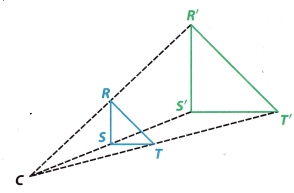A. Use a ruler to measure segments $$\overline{C R}, \overline{C R^{\prime}}, \overline{C S}, \overline{C S^{\prime}}, \overline{C T}$$ and $$\overline{C T^{\prime}}$$ to the nearest millimeter. Record the measurements and ratios in the table.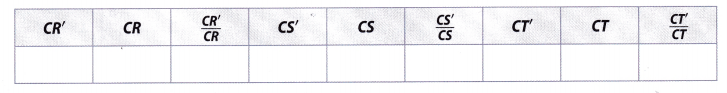B. Write a conjecture based on the ratios in the table.

C. Measure and record the corresponding side lengths of the triangles.D. Write a conjecture based on the ratios in the table.

E. Measure the corresponding angles and describe your results.

Reflect

Question 1.
Are triangles RSTand R’S’T’ similar? Why or why not?
Triangles RST and R’S’T’ are similar because their corresponding sides are proportional, and their corresponding angles are congruent.

Question 2.
Compare the orientation of a figure with the orientation of its dilation.
To determine the answer here, we will review the definition of a dilation.
Dilation is a transformation in which a certain shape changes its size, but shape and orientation stay the same. Therefore, we can claim that a certain figure and its dilation have the same orientation.

Explore Activity 2

Exploring Dilations on a Coordinate Plane

In this activity, you will explore how the coordinates of a figure on a coordinate plane are affected by a dilation.A. Complete the table. Record the x- and y-coordinates of the points in the two figures and the ratios of the x-coordinates and the y-coordinates.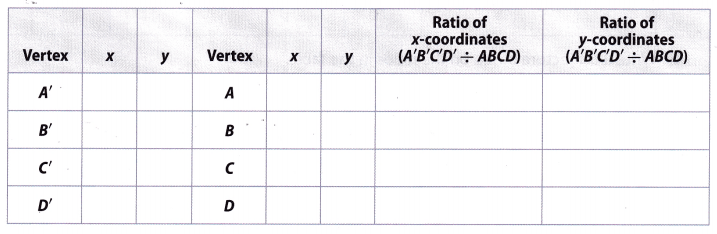B. Write a conjecture about the ratios of the coordinates of a dilation image to the coordinates of the original figure.

Reflect

Question 3.
In Explore Activity 1, triangle R’S’T’ was larger than triangle RST. How is the relationship between quadrilateral A’B’C’D’ and quadrilateral ABCD different?
Dilation is a transformation in which a certain object changes size depending on its scale factor. If the scale factor is bigger than 1, the image will be an enlargement and if the scale factor is less than 1, the picture will be a reduction.

Therefore, considering the fact that quadrilateral A’B’C’D’ is smaller than its original ABCD, we can claim that the scale factor was less than 1, so given dilation was a reduction.

Example 1

An art supply store sells several sizes of drawing triangles. All are dilations of a single basic triangle. The basic triangle and one of its dilations are shown on the grid. Find the scale factor of the dilation.STEP 1: Use the coordinates to find the lengths of the sides of each triangle.
Triangle ABC: AC = 2 CB = 3
Triangle A’B’C’: A’C’ = 4 C’B’ = 6
Since the scale factor is the same for all corresponding sides you can record just two pairs of side lengths. Use one pair as a check on the other.

STEP 2: Find the ratios of the corresponding sides.
$$\frac{A^{\prime} C^{\prime}}{A C}=\frac{4}{2}$$ = 2
$$\frac{C^{\prime} B^{\prime}}{C B}=\frac{6}{3}$$ = 3
The scale factor of the dilation is 2.

Reflect

Question 4.
Is the dilation an enlargement or a reduction? How can you tell?
Dilation is a transformation in which a certain object changes size depending on its scale factor. If the scale factor is bigger than 1, the image will be an enlargement and if the scale factor is less than 1, the picture will be a reduction.

Therefore, considering the fact that sides of a triangle A’B’G’ are bigger than the sides of the original image, we can conclude that the scale factor is bigger than 1.
Hence, given dilation is an enlargement.

Question 5.
Find the scale factor of the dilation.Use the coordinates to find the lengths of the sides of each rectangle:
Rectangle DEFG:
DE = FG = 6 ………………….. (1)
DG = EF = 4 ………………….. (2)
Rectangle D’E’F’G’ :
D’E’ = F’G’ = 3 ………………. (3)
D’G’ = E’F’ = 2 ……………… (4)
Find the ratios of the corresponding sides.
$$\frac{D^{\prime} G^{\prime}}{D G}=\frac{E^{\prime} F^{\prime}}{E F}=\frac{2}{4}=\frac{1}{2}$$ …………….. (5)
$$\frac{D^{\prime} E^{\prime}}{D E}=\frac{F^{\prime} G^{\prime}}{F G}=\frac{3}{6}=\frac{1}{2}$$ ……………… (6)

Use triangles ABC and A’B’C’ for 1-5. (Explore Activities 1 and 2, Example 1)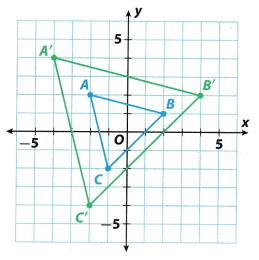Question 1.
For each pair of corresponding vertices, find the ratio of the x-coordinates and the ratio of the y-coordinates.
ratio of x-coordinates = ____________.
ratio of y-coordinates = ____________.
Firstly, we will have to identify x and y coordinates of triangles ABC and A’B’C’ by measuring how many units they are away from the origin.

Therefore, we can write coordinates as:
A(2, 2) A'(-4, 4)
B(2, 1) B'(4, 2)
C(-1, -2) C'(-2, -4)

To find the ratio of the x and y coordinates, we wilt firstly calculate the ratio between x coordinates for points ABC and A’B’C’ as following:Hence, we can conclude that the ratio of x coordinates is $$\frac{1}{2}$$.

Secondly, we wilL calculate the ratio of y coordinates using the same principle:Hence, we can conclude that the ratio of y coordinates is $$\frac{1}{2}$$.

Question 2.
I know that triangle A’B’C is a dilation of triangle ABC because the ratios of the corresponding
x-coordinates are ___________ and the ratios of the corresponding y-coordinates are ___________.
I know that triangle A’B’C’ is a dilation of triangle ABC because the ratios of the corresponding x-coordinates are equal and the ratios of the corresponding y-coordinates are equal.

Question 3.
The ratio of the lengths of the corresponding sides of triangle A’B’C’ and triangle ABC equals ___________.
To find the ratio of lengths, we first have to calculate the values of lengths using the given formula:
d = $$\sqrt{\left(x_{2}-x_{1}\right)^{2}+\left(y_{2}-y_{1}\right)^{2}}$$
where d is a distance between two points A(x1, y1) and B(x2, y2).

Using the formula given under (1), we can calculate the values of lengths for triangle A’B’C’ as following: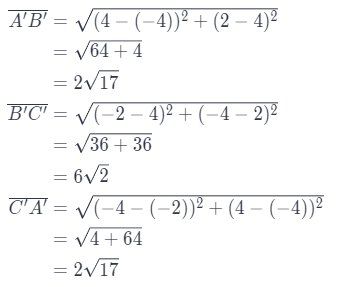Using the formula given under (1), we can calculate the values of lengths for triangle ABC as following:Using the obtained values, we can the ratio of the lengths as following: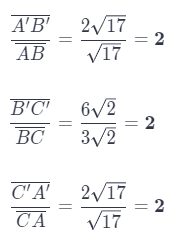Hence, we can conclude that ratio of the lengths is 2.

Question 4.
The corresponding angles of triangle ABC and triangle A’B’C’ are _________.
To determine the answer here, we will review the definition of congruent angles.
Two angles are congruent if their corresponding sides and angles have equal measure. Considering the fact that in the given exercise that is the case, we can claim that corresponding angles of triangles ABC and A’B’C’ are congruent.

Question 5.
The scale factor of the dilation is __________.
Considering the fact that scale factor of a dilation is defined as ratio of the lengths of corresponding sides, we will use the answer on Exercise 3.
Therefore, the scale factor is 2, meaning that triangle A’B’C’ is an enlargement of triangle ABC.

Essential Question Check-In

Question 6.
How can you find the scale factor of a dilation?
A scale factor described how much the figure is enlarged or reduced. It is the ratio of a length of the image to the corresponding length on the original figure.

By definition, to determine the scale factor of a dilation, we need to divide the length of the dilated image by the corresponding length of the original figure.

For 7-11, tell whether one figure is a dilation of the other or not. Explain your reasoning.

Question 7.
Quadrilateral MNPQ has side lengths: of 15 mm, 24 mm, 21 mm, and 18 mm. Quadrilateral M’N’P’Q’ has side lengths of 5 mm, 8 mm, 7 mm, and 4 mm.
Quadrilateral MNPQ has side lengths of 15 mm, 24 mm, 21 mm, and 18 mm. Quadrilateral M’N’P’Q’ has side lengths of 5 mm, 8 mm, 7 mm, and 4 mm. Find the ratios of the corresponding sides.
$$\frac{5}{15}=\frac{1}{3}$$ …………………….. (1)
$$\frac{8}{24}=\frac{1}{3}$$ …………………….. (2)
$$\frac{7}{21}=\frac{1}{3}$$ …………………….. (3)
$$\frac{4}{18}=\frac{2}{9}$$ …………………….. (4)
Since the ratios of the corresponding sides are not equal, the quadrilateraL MNPQ of M’N’P’Q’.

Question 8.
Triangle RST has angles measuring 38° and 75°. Triangle R’ST has angles measuring 67° and 38°.
Triangle RST has angles measuring 38° and 75°. We have to find third angle: 180° – 38° – 75° = 67°
Triangle R’S’T’ has angles measuring 67° and 38°. The measure of third angle is: 180° – 38° – 67° = 75°
Since the triangles have the same angle measures, the corresponding sides are proportional.

Question 9.
Two triangles, Triangle 1 and Triangle 2, are similar.
A dilation produces an image similar to the original figure, so Triangle 1 is a dilation of Triangle 2.

Question 10.
Quadrilateral MNPQ is the same shape but a different size than quadrilateral M’N’P’Q.
Quadrilateral MNPQ is the same like quadrilateral M’N’P’Q, so they are similar, and one is a dilation of the other.

Question 11.
On a coordinate plane, triangle UVW has coordinates U(20, -12), V(8, 6), and W(-24, -4). Triangle U’V’W’ has coordinates U'(15, -9), V'(6, 4.5), and W'(-18, -3).
Triangle UVW has coordinates U(20, -12), V(8, 6), and W(-24, -4). Triangle U’V’W’ has coordinates U'(15, -9), V'(6, 4.5), and W'(-18, 3) Find ratio of x-coordinates.
$$\frac{15}{20}=\frac{3}{4}$$ = (Divide fraction by 5) …………….. (1)
$$\frac{6}{8}=\frac{3}{4}$$ = (Divide fraction by 2) ……………… (2)
$$\frac{-18}{-24}=\frac{3}{4}$$ = (Divide fraction by -6) …………….. (3)
Find the ratio of y-coordinates
$$\frac{-9}{-12}=\frac{3}{4}$$ (Divide fraction by -3) ………………. (4)
$$\frac{4.5}{6}=\frac{1.5}{2}=\frac{3}{4}$$ (Divide fraction by 3 and then Expand the fraction with 2) ……………….. (5) (6)
$$\frac{-3}{-4}=\frac{3}{4}$$ = (Divide fraction by -1) ……………………… (7)
The ratio of the corresponding coordinates is equal, so the triangle UVW is a dilation of U’V’W’.

Complete the table by writing “same” or “changed” to compare the image with the original figure in the given transformation.

Question 12.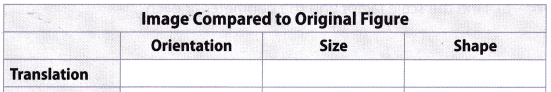The translation is a movement where we slide a geometric figure in any direction, meaning that the figure changes only its location, but orientation, size, and shape stay the same. Therefore, we can fill the given table as it is shown in the picture below.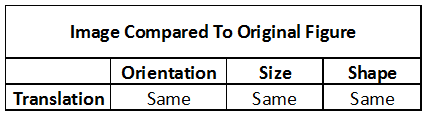Question 13.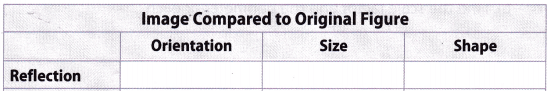Reflection is a movement where we flip a geometric figure regarding a certain line or a point, meaning that the figure changes its orientation, but size and shape stay the Same. Therefore, we can fill the given table as it is shown in the picture below.Question 14.Rotation is a movement where we rotate a geometric figure around any point for a certain amount of degrees, meaning that figure changes only its orientation, but size and shape stay the same Therefore, we can fill the given table as it is shown in the picture below.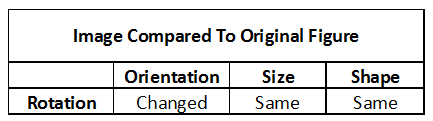Question 15.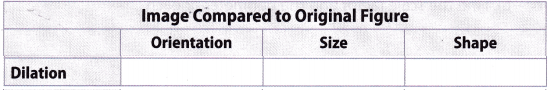Dilation is a transformation where we change a size of a given geometry object for a certain scale factor. Orientation and shape stay the same. Therefore, we can fill given table as it is shown in the picture below.Question 16.
Describe the image of a dilation with a scale factor of 1.
If the scale factor is 1, then the length of the corresponding sides is equal, so the image is congruent to the original figure.

Identify the scale factor used in each dilation.

Question 17.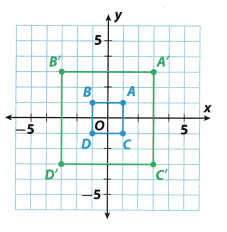Use the coordinates to find the lengths of the sides of each rectangle:
Rectangle ABCD: AB = BC = CD = AD = 2 (ABCD is a square)
A’B’C’D’: A’B’ = B’C’ = C’D’ = D’A’ = 6
Find the ratios of the corresponding sides.
$$\frac{A^{\prime} B^{\prime}}{A B}=\frac{6}{2}$$ (Substitute) (1)
= 3 (Calculate) (2)

Question 18.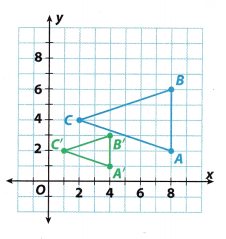To identify the scale factor, we firstly have to write coordinates of given points and then calculate the distance between them.

Coordinates of points ABC and A’B’C’ are:
A = (8, 2), B = (8, 6), C = (2, 4)
A’ = (4, 1), B’ = (4, 3), C’ = (1, 2)

To calculate the length of each side of triangle, we will use following formula:
d = $$\sqrt{\left(x_{2}-x_{1}\right)^{2}+\left(y_{2}-y_{1}\right)^{2}}$$
where distance between points A(x1, y1) and B(x2, y2) is d.

Using formula under (1), we can calculate length of each side in triangle ABC as following: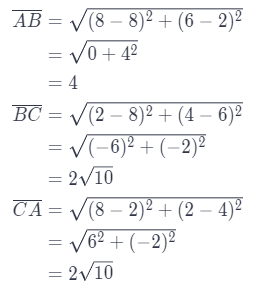Using formula under (1), we can calculate length of each side in triangle A’B’C’ as following:To calculate the scale factor of given dilation, we will calculate the ratio of belonging sides as following:Hence, we can conclude that scale factor used in given dilation is $$\frac{1}{2}$$.

H.O.T. Focus on Higher Order Thinking

Question 19.
Critical Thinking Explain how you can find the center of dilation of a triangle and its dilation.
Center of dilation is either a point or a plane from which we measure distance in dilation. We can find the center of dilation by drawing a line through the corresponding points of the picture before and after dilation. Those points intersect at the center of dilation.

Question 20.
Make a Conjecture
a. A square on the coordinate plane has vertices at (-2, 2), (2, 2), (2, -2), and (-2, -2). A dilation of the square has vertices at (-4, 4), (4, 4), (4, -4), and (-4, -4). Find the scale factor and the perimeter of each square.
A square on the coordinate pLane has vertices at (- 2, 2), (2, 2), (2, 2), and ( 2, 2). A dilation of the square has vertices at (- 4, 4), (4, 4), (4, 4), and (- 4, 4). Lets find the ratio of the corresponding coordinates.
$$\frac{-4}{-2}$$ = $$\frac{4}{2}$$ = 2
Length of one side of the original square is |2 (- 2)| = |2 + 2| = 4, so the perimeter P0 is
P0 = 4 ∙ 4 = 16
Length of one side of image square is |4 – (-4)| = |4 + 4| = 8, so the perimeter Pi is
Pi = 4 ∙ 8 = 32

b. A square on the coordinate plane has vertices at (-3, 3), (3, 3), (3, -3), and (-3, -3). A dilation of the square has vertices at (-6, 6), (6, 6), (6, -6), and (-6, -6). Find the scale factor and the perimeter of each square.
$$\frac{-6}{-3}$$ = $$\frac{6}{3}$$ = 2